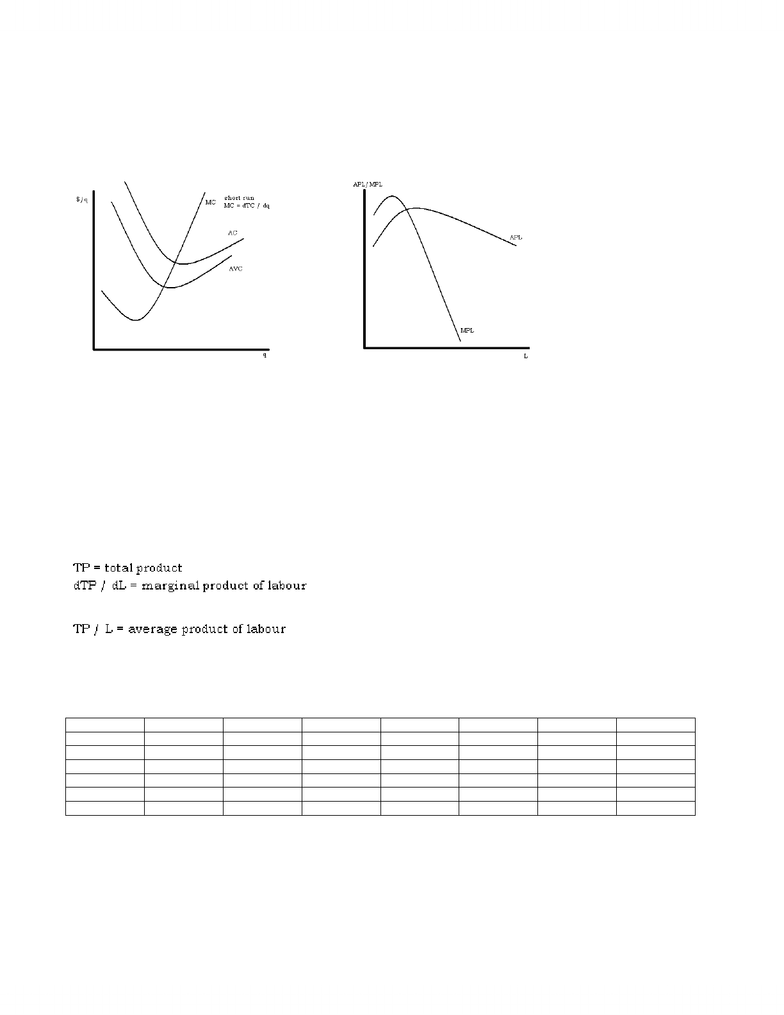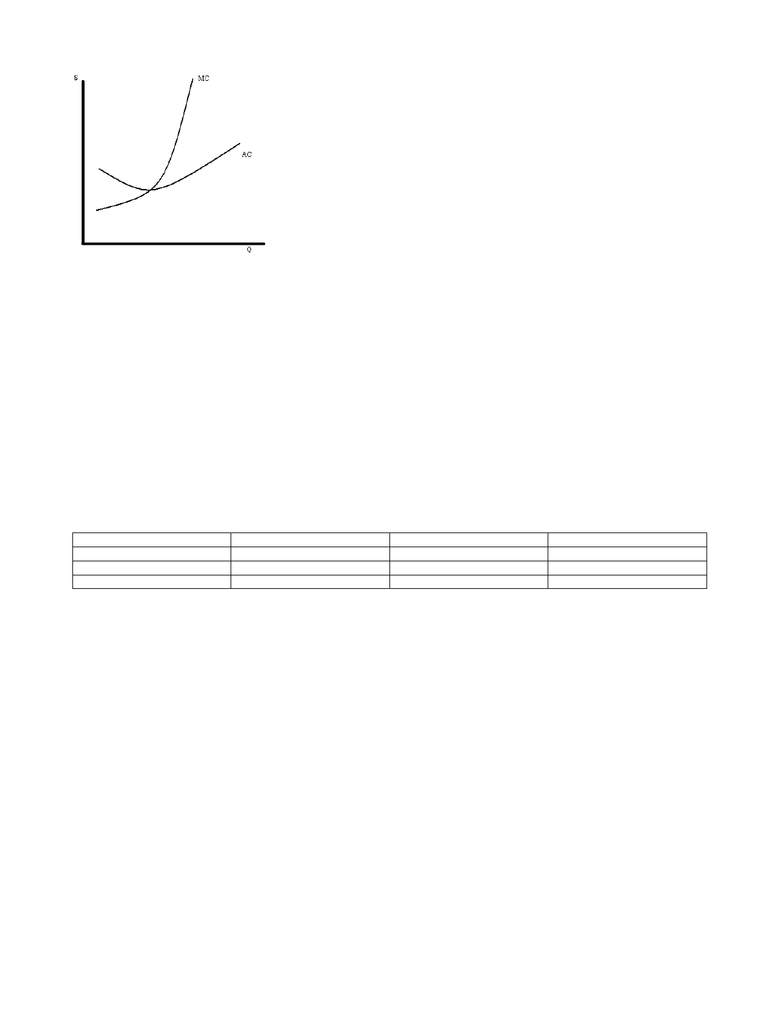# MGEA02H3 Lecture Notes - Lecture 5: Marginal Product

35 views5 pagesECMA04H
Week 5
We will finish up Elasticity and Excess Burden of an Excise Tax and then move on to…
Production, Productivity and Costs
Production, productivity and costs
Basic objective….understand diminishing marginal productivity and the shapes of typical cost curves in short run.
Why?: to understand the supply curve. Decisions by firms about how much to produce/supply.
We assume that the objective of firms is to maximize profits!
They hire labour and purchase (or rent) capital equipment and other inputs. These inputs combine together to produce output. Output is
sold to earn a profit (or loss…./)
A simple story to motivate what this week is all about:
Imagine that you and 4 other friends are in a lawn mowing business. You have only one lawnmower (fixed amount of capital). The
following is the “production function”
1 workers + 1 lawnmower = 5 lawns per day
2 workers + 1 lawnmower = 9 lawns per day
3 workers + 1 lawnmower = 12 lawns per day
4 workers + 1 lawnmower = 14 lawns per day
5 workers + 1 lawnmower = 15 lawns per day
The “marginal product of labour” (additional output from the additional worker) is 5 lawns for the first worker, 4 more for the
second, 3 more for the third, 2 more for the fourth, and 1 more for the final worker
The “average product of labour is 5 lawns per worker when there is 1 worker, 4.5 lawns per worker when there are 2 workers, 4 lawns
per worker when there are 3 workers, 3.5 lawns per worker when there are 4 workers, and 3 lawns per worker when there are 5 workers
If the rental on the lawnmower costs \$30 per day (cost of capital equipment) (fixed cost) and the wage paid to each worker is \$50 per day
(variable cost), then the cost of cutting the first 5 lawns is \$30 + \$50 = \$80. The cost of cutting 9 lawns per day is \$30 + 2*\$50 = \$130.
The cost of cutting 12 lawns is \$30 + 3*\$50 = \$180. The cost of cutting 14 lawns is \$30 + 4*\$50 = \$230. And the cost of cutting 15 lawns
per day is \$30 + 5*\$50 = \$280.
We can calculate the average cost per lawn, and the marginal cost per lawn (change in costs as output changes).
L K Q = TP MPL APL TC AC MC
0 1 0 0 0 \$30 Ø
1 1 5 5 5 \$80 \$16 \$10
2 1 9 4 4.5 \$130 \$14.44 \$12.50
3 1 12 3 4 \$180 \$15 \$16.67
4 1 14 2 3.5 \$230 \$16.43 \$25
5 1 15 1 3 \$280 \$18.67 \$50
L = labour K = fixed costs
Q = output MPL = marginal product of labour
APL = average product of labour TC = total costs
AC = TC / Q = average cost per unit of output MC = dTC / dq = marginal cost
Draw the average cost and marginal cost curves. As long as there are fixed inputs (e.g., capital equipment) in the short run, the AC and
MC curves will have these shapes.
www.notesolution.com
Unlock document

This preview shows pages 1-2 of the document.
Unlock all 5 pages and 3 million more documents.Most firms, most of the time, have no control over the production technology available. They adopt existing technology.
We write this as q = f(K,L)
K = inputs of capital (machinery, buildings, etc)
L = inputs of labour (hours of standardized worker)
An example of a production function:
q = KALB
(called “Cobb-Douglas” function)
even simpler version of Cobb-Douglas function is to choose
A = B = 0.5
[Note: A and B are parameters of the Cobb-Douglas function]
So, we have q = (KL)0.5
Now define time period: short run, long run, very long run
Short run = period too short for firms to change production capacity (K)
Long run = period long enough for firms to change plant capacity, and for new firms to enter industry (or exit)
Very long run = period long enough for technology to change
SR (K is fixed in amount)
technology capital labour
short run fixed fixed variable
long run fixed variable variable
very long run variable variable variable
e.g., long run: q = (KL)0.5
in short run, we would have to assume K = constant
e.g., K = 9, so q = 3L0.5 (short run production function)
Now define
marginal product of labour = MPL = dq/dL
In example we used earlier
K = 9, so q = 3L0.5
we get MPL = dq/dL = 1.5L-0.5 = 1.5/L0.5
We assume that dMPL/dL < 0
This is called diminishing marginal product
“Law of Diminishing Returns” (or law of diminishing marginal product, or law of variable proportions)
Thomas Malthus (Malthusian economics)
NOW we go to the next stage: we want to link production to costs
TC = PKK + PLL
PKK = (rental) cost of capital equipment
PK = rental price of capital equipment (fixed)
K = amount of capital equipment (in short run, fixed)
PLL = cost of labour
PL = price of labour (wages) (fixed)
L = number of units of labour (in short run, variable)
In short run, K fixed and L variable, so
PKK = fixed cost
www.notesolution.com
Unlock document

This preview shows pages 1-2 of the document.
Unlock all 5 pages and 3 million more documents.

## Get access

\$10 USD/m
Billed \$120 USD annuallyHomework Help
Study Guides
Textbook Solutions
Class Notes
Textbook Notes
Booster Class